NEWS CATEGORY

• ### 采购指南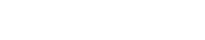MORE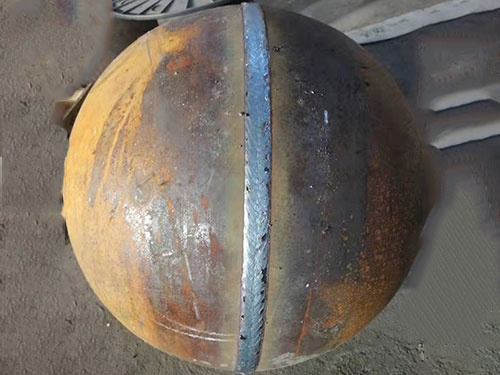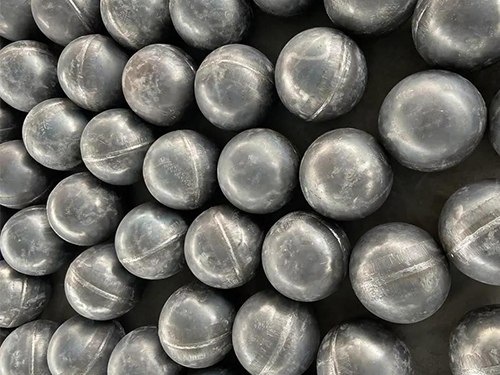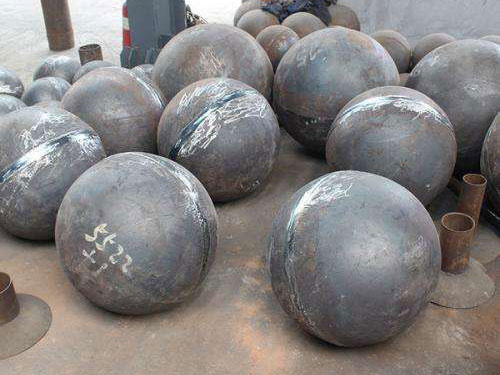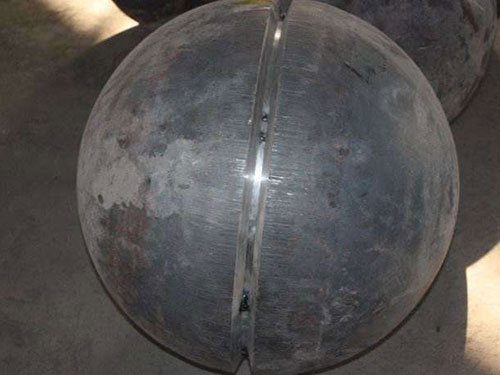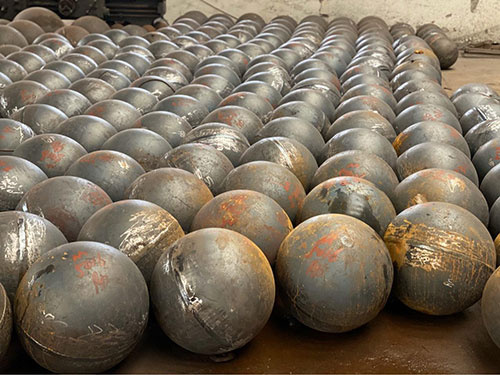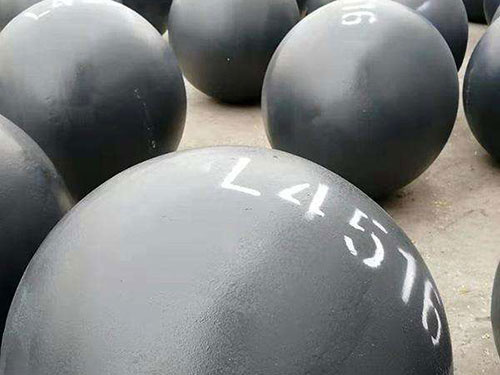# 椭圆封头的厚度计算

2013-08-28 09:36:55

KpDi
计算厚度δ=----------------=4.73mm
2[σ]tΦ-0.5pc
计算厚度δd=δ + C2=4.73+1=5.73mm
考虑标准椭圆封头   厚度δe应不小于封头内径Di的0.15%，   厚度δe=0.15%Di=6mm
δe>δd、C1=0、C2=1、名义厚度δn=δe+C1+C2=6+0+1=7mm
考虑钢材标准规格厚度作了上浮1mm的厚度次设计圆整值△1=1，故取δn=8mm。
根据   封头制造厂技术资料Di=4000、δn=8封头加工减薄量C3=1.5mm，经厚度次圆整值△2=0.5。
如要求封头成形厚度不得小于名义厚度δn减钢板负偏差C1，则投料厚度：
δs=δn+C1+C3+△2=8+0+1.5+0.5=10mm，而成形后的小厚度为8.5mm。如采用封头成形厚度不小于设计厚度δd（应取δe值），则投料厚度：δs=δd（δe）+C3+△2=8mm，而成形后的小厚度为6.5mm、且大于   厚度δe、大于设计厚度δd和计算厚度δ。

• 网站首页

• ## 联系方式

• ### 翻边